# Python：游戏：五子棋之人机对战

• A+

### 开端

#### 画棋子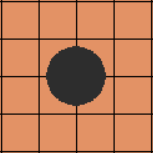`pygame.draw` 中有画抗锯齿直线的函数
`aaline`，但是并没有
`aacircle` 这样的函数来画一个抗锯齿的圆。

``col = (255, 0, 0)surf.fill((255, 255, 255))pygame.gfxdraw.aacircle(surf, x, y, 30, col)pygame.gfxdraw.filled_circle(surf, x, y, 30, col)``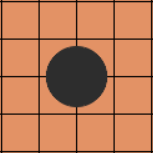#### 落子

``    while True:        for event in pygame.event.get():            if event.type == QUIT:                sys.exit()            elif event.type == MOUSEBUTTONDOWN:                pressed_array = pygame.mouse.get_pressed()                if pressed_array:  # 鼠标左键点击                    mouse_pos = pygame.mouse.get_pos()                    click_point = _get_clickpoint(mouse_pos)``

### 胜利判定

``Chessman = namedtuple('Chessman', 'Name Value Color')Point = namedtuple('Point', 'X Y')BLACK_CHESSMAN = Chessman('黑子', 1, (45, 45, 45))WHITE_CHESSMAN = Chessman('白子', 2, (219, 219, 219))offset = [(1, 0), (0, 1), (1, 1), (1, -1)]class Checkerboard:    def __init__(self, line_points):        self._line_points = line_points        self._checkerboard = [ * line_points for _ in range(line_points)]    def _get_checkerboard(self):        return self._checkerboard    checkerboard = property(_get_checkerboard)    # 判断是否可落子    def can_drop(self, point):        return self._checkerboard[point.Y][point.X] == 0    def drop(self, chessman, point):        """        落子        :param chessman: 黑子/白子        :param point:落子位置        :return:若该子落下之后即可获胜，则返回获胜方，否则返回 None        """        print(f'{chessman。Name} ({point.X}, {point.Y})')        self._checkerboard[point.Y][point.X] = chessman.Value        if self._win(point):            print(f'{chessman。Name}获胜')            return chessman    # 判断是否赢了    def _win(self, point):        cur_value = self._checkerboard[point.Y][point.X]        for os in offset:            if self._get_count_on_direction(point, cur_value, os, os):                return True    def _get_count_on_direction(self, point, value, x_offset, y_offset):        count = 1        for step in range(1, 5):            x = point.X + step * x_offset            y = point.Y + step * y_offset            if 0 <= x < self._line_points and 0 <= y < self._line_points and self._checkerboard[y][x] == value:                count += 1            else:                break        for step in range(1, 5):            x = point.X - step * x_offset            y = point.Y - step * y_offset            if 0 <= x < self._line_points and 0 <= y < self._line_points and self._checkerboard[y][x] == value:                count += 1            else:                break        return count >= 5``

### 电脑落子

#### 连五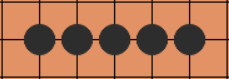#### 活四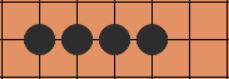#### 冲四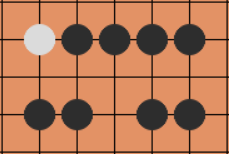#### 活三、跳活三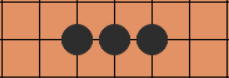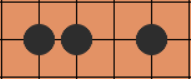#### 眠三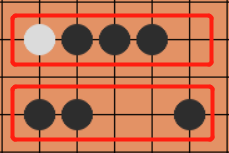#### 打分机制

• 首先，连五肯定是不存在的，出现连五胜负已分，所以只要棋局还在进行中，就不会出现连五。那么，什么优先级最高？自然就是活四了。
• 其次是对方的“四”，对方活四，你防不防都一样输了，对方冲四，你就必须防守。
• 再次是我方的活三或冲四，活三跟冲四其实是一个级别的，对方必须防守。
• 再次是对方的活三或冲四。

• 相同的棋形,我方优于对方。
• 冲四跟活三一个级别，眠三跟活二一个级别。
• 如果中间有空格的话，肯定是要比没空格的略微低级一点，但不至于降级。